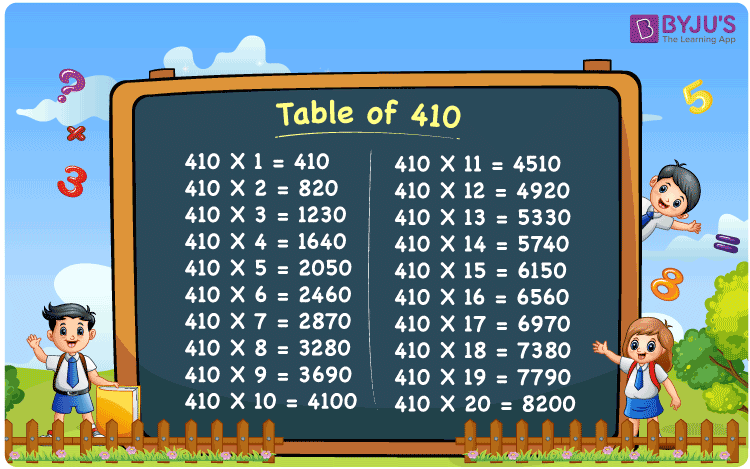Checkout JEE MAINS 2022 Question Paper Analysis : Checkout JEE MAINS 2022 Question Paper Analysis :

# Table of 410

The Table of 410 is provided in the table grid form to help students quickly check the values of multiples of 410. A printable PDF of the multiplication table of 410 is provided so that students can download this to memorise the table of 410.

## Table of 410 Chart

The 410 times table is presented as an image that can be downloaded and used as flashcards to memorise the multiplication table of 410. These flashcards are handy for quick mental maths calculations, and in solving various questions.## What is the 410 times table?

The 410 times table is the list of all multiples of 410. Any natural number multiplied by 410 is the multiple of 410. The multiplication table of 410 can be represented in both multiplication and addition form. The below table lists the multiples of 410 in both multiplication and addition form.

 410×1 = 410 410 410×2 = 820 410 + 410 = 820 410×3 = 1230 410 + 410 + 410 = 1230 410×4 = 1640 410 + 410 + 410 + 410 = 1640 410×5 = 2050 410 + 410 + 410 + 410 + 410 = 2050 410×6 = 2460 410 + 410 + 410 + 410 + 410 + 410 = 2460 410×7 = 2870 410 + 410 + 410 + 410 + 410 + 410 + 410 = 2870 410×8 = 3280 410 + 410 + 410 + 410 + 410 + 410 + 410 + 410 = 3280 410×9 = 3690 410 + 410 + 410 + 410 + 410 + 410 + 410 + 410 + 410 = 3690 410×10 = 4100 410 + 410 + 410 + 410 + 410 + 410 + 410 + 410 + 410 + 410 = 4100

## Multiplication Table of 410

Given below is the table of 410 listed up to 20 multiples.

 410 × 1 = 410 410 × 2 = 820 410 × 3 = 1230 410 × 4 = 1640 410 × 5 = 2050 410 × 6 = 2460 410 × 7 = 2870 410 × 8 = 3280 410 × 9 = 3690 410 × 10 = 4100 410 × 11 = 4510 410 × 12 = 4920 410 × 13 = 5330 410 × 14 = 5740 410 × 15 = 6150 410 × 16 = 6560 410 × 17 = 6970 410 × 18 = 7380 410 × 19 = 7790 410 × 20 = 8200

## Solved Examples on the Table of 410

Example 1:

What is the difference between the 12th multiple and the 20th multiple of 410?

Solution:

Using the multiplication table of 410

The 12th multiple of 410 = 4920

The 20th multiple of 410 = 8200

Difference between the 12th multiple and 20th multiple = 8200 – 4920  = 3280.

## Frequently Asked Questions on Table of 410

### What is the table of 410?

The table of 410 is the list of all of multiples of 410 starting from 410 × 1 = 410, 410 × 2 = 810, 410 × 3 = 1230, 410 × 4 = 1640, 410 × 5 = 2050 and so on.

### Which times table has 410?

410 comes in the table of 1, 2, 5, 10, 41, 82, 205 and 410.

### What is 15 times 410?

15 times 410 is 6150.